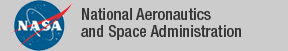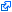### Top File of PVS metric_space Library

```
%------------------------------------------------------------------------------
% Metric Spaces
%
%     Author: David Lester, Manchester University
%
% All references are to WA Sutherland "Introduction to Metric and
% Topological Spaces", OUP, 1981
%
%     Version 1.0            17/08/07  Initial Version
%
% Note that an alternate version of metric spaces is available in the analysis
% library.  See
%
%    metric_spaces[T:TYPE+,d:[T,T->nnreal]]: THEORY
%    BEGIN
%
%      ASSUMING IMPORTING metric_spaces_def[T,d]
%          fullset_metric_space: ASSUMPTION metric_space?[T,d](fullset[T])
%      ENDASSUMING
%
% This version does not use the topology library.
%------------------------------------------------------------------------------

top: THEORY

BEGIN

IMPORTING
% Extra results
countable_cross,   % the cross product of two countable sets is countable

% Basic properties
metric_def,        % Definition of metric
metric_space_def,  % Basic properties of metric spaces
metric_space,      % Deeper results
submetric_def,     % metrics for sub-spaces
metric_subspace,   % subspaces of metric spaces
complete_product,  % products of complete metrics

% Continuity in metric spaces
metric_continuity, % Continuity expressed using metrics (epsilon/delta)
composition_continuous,
% composition is continuous
composition_uniform_continuity,
% composing uniform continuous functions

% Convergence properties
convergence_aux,   % Properties of convergence in metric spaces

% real topology results
real_topology,     % The topology of the reals
heine_borel_scaf,  % Foundations for ...
heine_borel,       % Heine-Borel
euclidean,         % Topology of R^n (Vector[n])
real_continuity,   % Continuity of functions [T->real]
%%%%%%%%%%%%%% RWB %%%%%%%%%%%%% <. %%%%%%%%%%%%%
The tagidentifies links that are outside the NASA domain